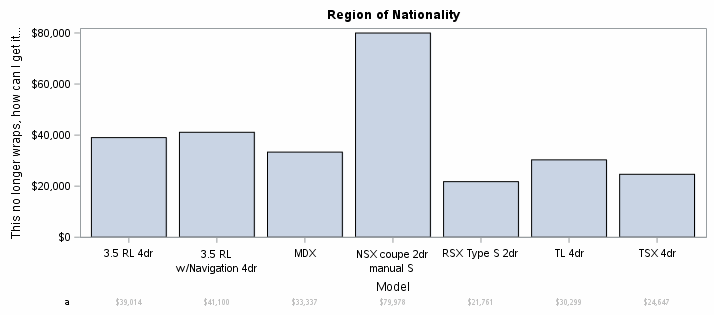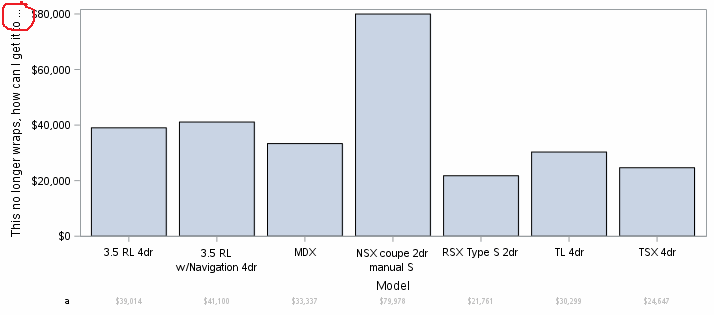## How to wrap yaxis title when using an xaxistable in proc sgplot

Hi - Is there a way to wrap a y-axis title when using xaxistable (to get around at present I am using labelpos=top, but would prefer it at the default for consistency with other charts).

Eg. of problem the following code :

``````proc sgplot data=sashelp.cars(where=(make = 'Acura'));
vbar model/response=invoice;
yaxis label= "This no longer wraps, how can I get it to wrap?";
xaxistable invoice /label="a" valueattrs= (color=liggr) labelattrs=(size=8pt);
run;``````

returns the following:1 ACCEPTED SOLUTION

Accepted Solutions

## Re: How to wrap yaxis title when using an xaxistable in proc sgplot

It will be the yaxis in your case.

HTH.

4 REPLIES 4

## How to wrap yaxis title when using an xaxistable in proc sgplot

Hi - Is there a way to wrap a y-axis title when using xaxistable (to get around at present I am using labelpos=top, but would prefer it at the default for consistency with other charts).

Eg. of problem the following code :

``````proc sgplot data=sashelp.cars(where=(make = 'Acura'));
vbar model/response=invoice;
yaxis label= "This no longer wraps, how can I get it to wrap?";
xaxistable invoice /label="a" valueattrs= (color=liggr) labelattrs=(size=8pt);
run;``````

returns the following:## Re: How to wrap yaxis title when using an xaxistable in proc sgplot

It will be the yaxis in your case.

HTH.Jay54
Meteorite | Level 14

## Re: How to wrap yaxis title when using an xaxistable in proc sgplot

Another way, not mentioned in the linked article is to place the xaxistable inside the plot area.  Set LOCATION=INSIDE on the xAxisTable.

``xaxistable invoice /label="a" valueattrs= (color=liggr) labelattrs=(size=8pt) location=inside;``

## Re: How to wrap yaxis title when using an xaxistable in proc sgplot

Thank-you - that also works.
Discussion stats
• 4 replies
• 3658 views
• 5 likes
• 3 in conversation